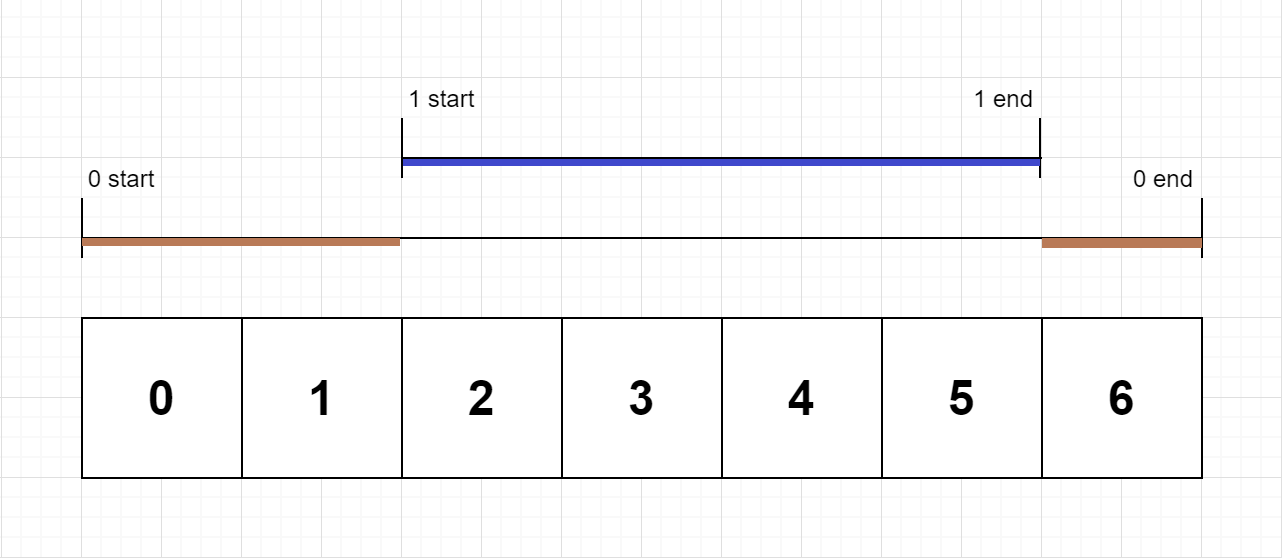### LeetCode• ㊗️
• 大家
• offer
• 多多！

## Problem

On a single-threaded CPU, we execute a program containing n functions. Each function has a unique ID between 0 and n-1.

Function calls are stored in a call stack: when a function call starts, its ID is pushed onto the stack, and when a function call ends, its ID is popped off the stack. The function whose ID is at the top of the stack is the current function being executed. Each time a function starts or ends, we write a log with the ID, whether it started or ended, and the timestamp.

You are given a list logs, where logs[i] represents the ith log message formatted as a string “{function_id}:{“start” | “end”}:{timestamp}”. For example, “0:start:3” means a function call with function ID 0 started at the beginning of timestamp 3, and “1:end:2” means a function call with function ID 1 ended at the end of timestamp 2. Note that a function can be called multiple times, possibly recursively.

A function’s exclusive time is the sum of execution times for all function calls in the program. For example, if a function is called twice, one call executing for 2 time units and another call executing for 1 time unit, the exclusive time is 2 + 1 = 3.

Return the exclusive time of each function in an array, where the value at the $i^th$ index represents the exclusive time for the function with ID i.

Example 1:Input: n = 2, logs = ["0:start:0","1:start:2","1:end:5","0:end:6"]
Output: [3,4]
Explanation:
Function 0 starts at the beginning of time 0, then it executes 2 for units of time and reaches the end of time 1.
Function 1 starts at the beginning of time 2, executes for 4 units of time, and ends at the end of time 5.
Function 0 resumes execution at the beginning of time 6 and executes for 1 unit of time.
So function 0 spends 2 + 1 = 3 units of total time executing, and function 1 spends 4 units of total time executing.


Example 2:

Input: n = 1, logs = ["0:start:0","0:start:2","0:end:5","0:start:6","0:end:6","0:end:7"]
Output: 
Explanation:
Function 0 starts at the beginning of time 0, executes for 2 units of time, and recursively calls itself.
Function 0 (recursive call) starts at the beginning of time 2 and executes for 4 units of time.
Function 0 (initial call) resumes execution then immediately calls itself again.
Function 0 (2nd recursive call) starts at the beginning of time 6 and executes for 1 unit of time.
Function 0 (initial call) resumes execution at the beginning of time 7 and executes for 1 unit of time.
So function 0 spends 2 + 4 + 1 + 1 = 8 units of total time executing.


Example 3:

Input: n = 2, logs = ["0:start:0","0:start:2","0:end:5","1:start:6","1:end:6","0:end:7"]
Output: [7,1]
Explanation:
Function 0 starts at the beginning of time 0, executes for 2 units of time, and recursively calls itself.
Function 0 (recursive call) starts at the beginning of time 2 and executes for 4 units of time.
Function 0 (initial call) resumes execution then immediately calls function 1.
Function 1 starts at the beginning of time 6, executes 1 units of time, and ends at the end of time 6.
Function 0 resumes execution at the beginning of time 6 and executes for 2 units of time.
So function 0 spends 2 + 4 + 1 = 7 units of total time executing, and function 1 spends 1 unit of total time executing.


Example 4:

Input: n = 2, logs = ["0:start:0","0:start:2","0:end:5","1:start:7","1:end:7","0:end:8"]
Output: [8,1]


Example 5:

Input: n = 1, logs = ["0:start:0","0:end:0"]
Output: 


Constraints:

• 1 <= n <= 100
• 1 <= logs.length <= 500
• 0 <= function_id < n
• $0 <= timestamp <= 10^9$
• No two start events will happen at the same timestamp.
• No two end events will happen at the same timestamp.
• Each function has an “end” log for each “start” log.

## Code

class Solution {
class Log {
int id;
boolean isStart;
int time;

Log(String log) {
String[] content = log.split(":");
id = Integer.parseInt(content);
isStart = content.equals("start");
time = Integer.parseInt(content);
}
}

public int[] exclusiveTime(int n, List<String> logs) {
int[] res = new int[n];
Stack<Integer> stack = new Stack<>();
int lastEndTime = 0;

for (String log : logs) {
Log logObj = new Log(log);

if (logObj.isStart) {
if (!stack.isEmpty()) {
int id = stack.peek();
res[id] += logObj.time - lastEndTime;
}
lastEndTime = logObj.time;
stack.push(logObj.id);
} else {
int id = stack.pop();
res[id] += logObj.time - lastEndTime + 1;
lastEndTime = logObj.time + 1;
}
}

return res;
}
}